# Watts / Volts / Amps / Ohms calculator

Watts (W) - volts (V) - amps (A) - ohms (Ω) calculator.

Calculates dc power / voltage / current / resistance.

Enter 2 values to get the other values and press the Calculate button:

Ohm's law calculator ►

### Ohms calculations

So The resistance R in ohms (Ω) is equal to the voltage V in volts (V) divided by the current I in amps (A).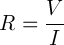So The resistance R in ohms (Ω) is equal to the squared voltage V in volts (V) divided by the power P in watts (W).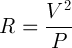So The resistance R in ohms (Ω) is equal to the power P in watts (W) divided by the squared current I in amps (A).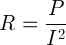### Amps calculations

So The current I in amps (A) is equal to the voltage V in volts (V) divided by the resistance R in ohms (Ω).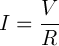So The current I in amps (A) is equal to the power P in watts (W) divided by the voltage V in volts (V).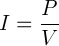So The current I in amps (A) is equal to the square root of the power P in watts (W) divided by the resistance R in ohms (Ω).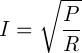### Volts calculations

So The voltage V in volts (V) is equal to the current I in amps (A) times the resistance R in ohms (Ω).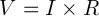So The voltage V in volts (V) is equal to the power P in watts (W) divided by the current I in amps (A).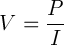So The voltage V in volts (V) is equal to the square root of the power P in watts (W) times the resistance R in ohms (Ω):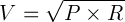### Watts calculation

So The power P in watts (W) is equal to the voltage V in volts (V) times the current I in amps (A).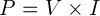So The power P in watts (W) is equal to the squared voltage V in volts (V) divided by the resistance R in ohms (Ω).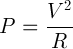So The power P in watts (W) is equal to the squared current I in amps (A) times the resistance R in ohms (Ω).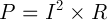Ohm's law calculator ►

## Features of Watts / Volts / Amps / Ohms Calculator

Our Watts / Volts / Amps / Ohms converter allows the users to Calculate Watts / Volts / Amps / Ohms. Some of the prominent features of this utility are explained below.

### No Registration

You don’t need to go through any registration process to use the Watts / Volts / Amps / Ohms converter. Using this utility, you can convert Watts / Volts / Amps / Ohms as many times as you want for free.

#### Fast conversion

This Watts / Volts / Amps / Ohms Calculator offers users the fastest conversion. Once the user enters the  Watts / Volts / Amps / Ohms values ​​in the input field and clicks the Convert button, the utility will start the conversion process and return the results immediately.

#### Saves Time and Effort

The manual procedure of Calculator Watts / Volts / Amps / Ohms isn’t an easy task. You must spend a lot of time and effort to complete this task. The Watts / Volts / Amps / Ohms Calculator allows you to complete the same task immediately. You will not be asked to follow manual procedures, as its automated algorithms will do the work for you.

##### Accuracy

Despite investing time and effort in manual Calculation, you might not be able to get your hands on accurate results. Not everyone is good at solving math problems, even if you think you're a pro, there's still a good chance you'll get in accurate results. This situation can be smartly handled with the help of a Watts / Volts / Amps / Ohms Calculator. You will be provided with 100% accurate results by this online tool.

##### Compatibility

The online Watts / Volts / Amps / Ohms converter perfectly works on all operating systems. Whether you have a Mac, iOS, Android, Windows, or Linux device, you can easily use this online Tool without facing any hassle.

###### 100% Free

You don't need to go through any registration process to use this Watts / Volts / Amps / Ohms Calculator. You can use this utility for free and do unlimited Watts / Volts / Amps / Ohms Convert without any limitations.